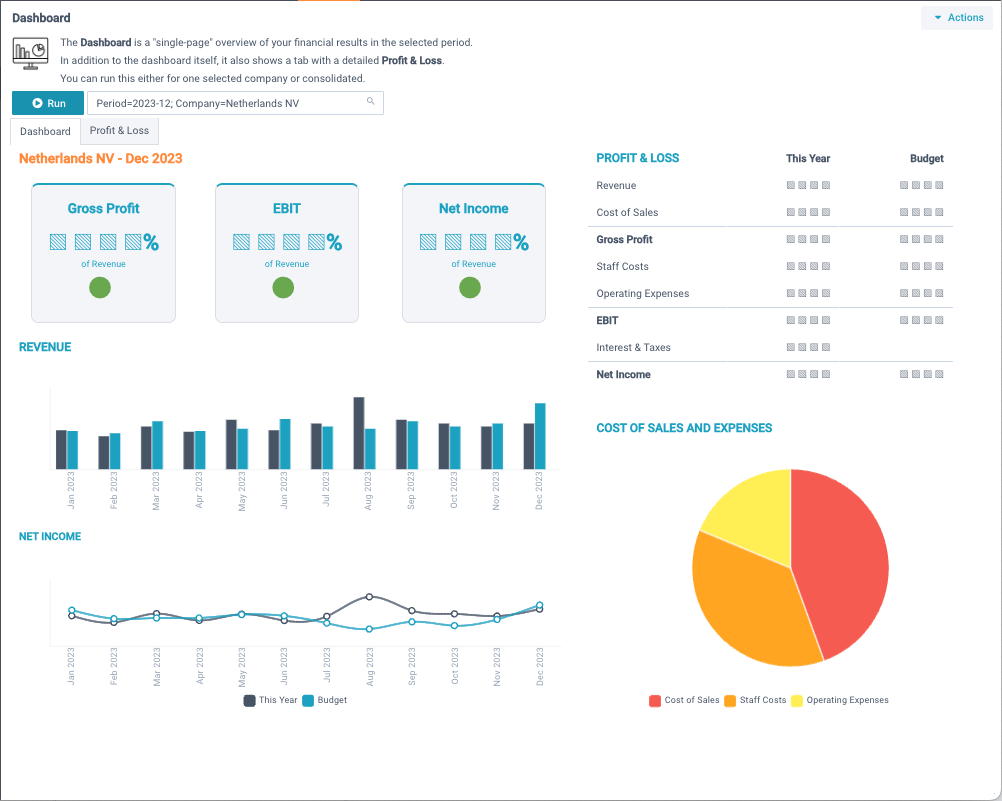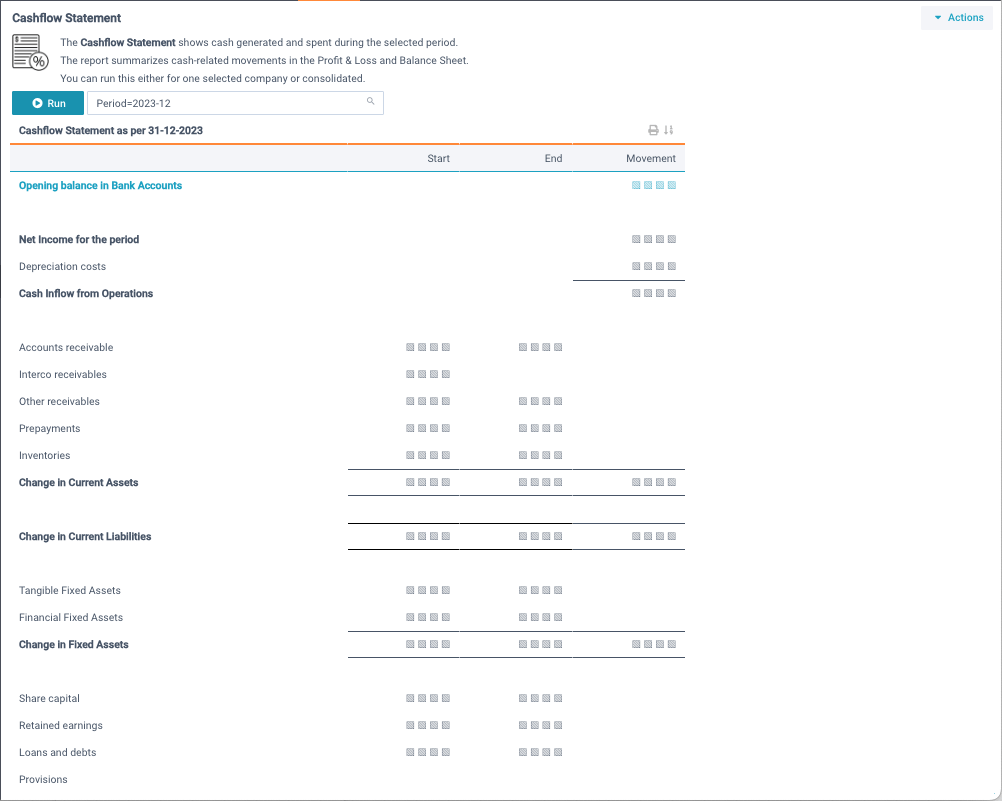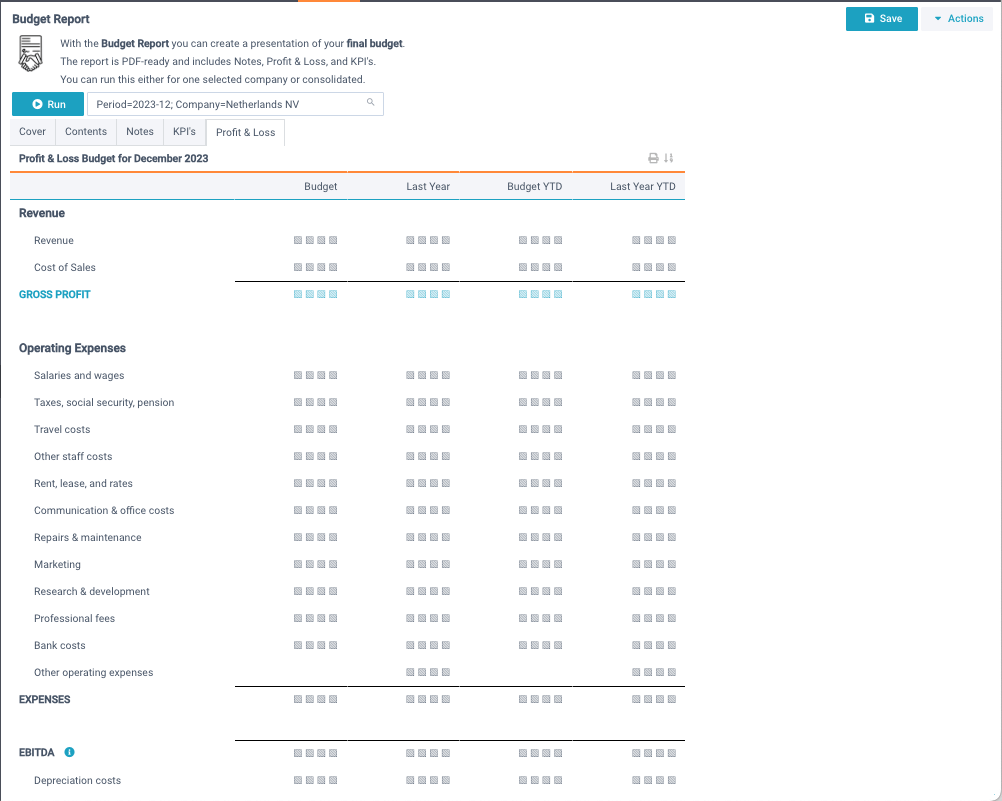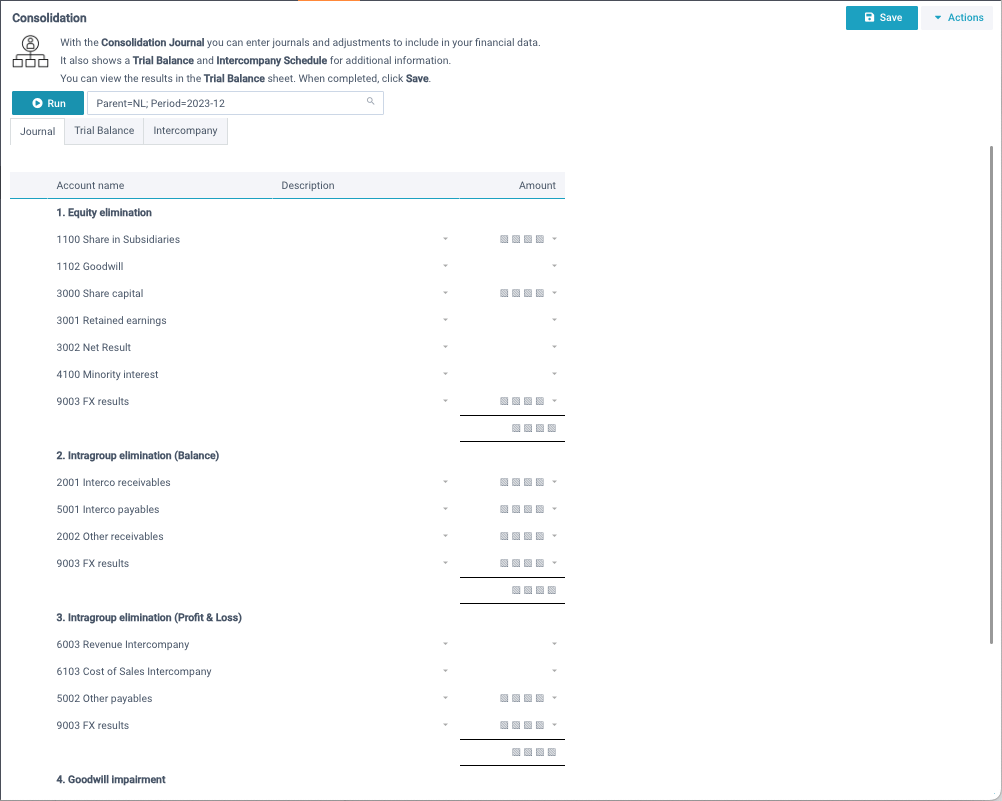# The demo models explained

Models are configurable objects that combine data queries with spreadsheet-like templates, enabling you to build advanced reporting and interactive planning models. You can use all common spreadsheet features such as calculations, formulas, functions, texts, cell formats, ranges etc. And much more!

All demo models are defined on the data set Financial data which contains actuals and budget data. This data set is linked to 2 other data sets: Companies and Accounts which brings up information about the company structure and the chart of accounts.

## Management reportIn this model we defined a data query to read data from your data sets into memory, which enables you to use cell functions to insert data into individual cells in your model.

The data query retrieves trial balance information with 6 columns: Class, Category, Account, Period amount, Previous period amount, and Year-to-date amount. In Define model, you can select this query and click the Define or Preview button to see how it is defined.

We then used the function DSUM to get the sum of values of all rows in the query that meet a given criteria. For example, Gross profitability in cell B2 is calculated as follows:

=DSUM("Gross margin", 1, 4, 1) / DSUM("Revenue", 1, 4, 2)

So ... let's explain that:

• From query 1, it takes the SUM of all amounts in column 4 where column 1 matches "Gross margin".
• From query 1, it takes the SUM of all amounts in column 4 where column 1 matches "Revenue".
• And divide the 2 amounts.
In other words: Gross profitability = Gross margin / Revenue. That makes sense?
We used cell formatting to show the result as a percentage.

All other formulas in this model follow the same principle and insert different amounts from the query into the model. The model also contains normal cell calculations such as in cell D2: =B2-C2. The logic of the KPI calculation is determined by the formulas, and you can change them to anything you want. The model only contains logic, the numbers are always inserted realtime.

## Cashflow StatementIn this model we defined a data query to read data from your data sets into memory, which enables you to use cell functions to insert data into individual cells in your model.

The data query retrieve trial balance information with 5 columns: Class, Category, Account, Period amount, and Previous period amount. In Define model, you can select this query and click the Define or Preview button to see how it is defined.

We then used the function DSUM to get the sum of values of all rows in the query that meet a given criteria. For example, Net income in cell D2 is calculated as follows:

=DSUM("Gross margin", 1, 4, 1) - DSUM("Expenses", 1, 4, 1) - DSUM("Finance cost", 1, 4, 1)

So ... let's explain that:

• From query 1, it takes the SUM of all amounts in column 4 where column 1 matches "Gross margin".
• From query 1, it takes the SUM of all amounts in column 4 where column 1 matches "Expenses", and substracts that from above.
• From query 1, it takes the SUM of all amounts in column 4 where column 1 matches "Finance costs", and substracts that from above.
In other words: Net income = Gross margin - Expenses - Finance costs. That makes sense?

All other formulas in this model follow the same principle and insert different amounts from the query into the model. The model also contains normal cell calculations such as in cell D13: =B13-C13. The logic of the cashflow statement is determined by the formulas, and you can change them to anything you want. The model only contains logic, the numbers are always inserted realtime.

## Budget modelIn this model, we defined a report query to insert last year's Profit & Loss data into the model, and a import query to save the calculated budget data back into your data sets. In Define model, you can select a query and click the Define or Preview button to see how it is defined.

The sheet Parameters enables the user to key in sales, production, and headcount parameters, which are then calculated into amounts. The sheet Profit & Loss contains formulas that use those calculated amounts, and enables the user to enter adjustments to the calculated amounts and select from defined planners that spread the total amounts over 12 months. We used the PLANNER function for this.

All this is done using normal spreadsheet-like functions and formulas. In Define model, you can navigate to the various cells to see the formulas.

By default, all cells in XLReporting are locked when you run a model, apart from cells where you have defined so-called cell editors. So we defined cell editors where we want users to key in values. Using normal cell formatting, we also gave those cells a green background.

The logic of the budget model is determined by the formulas, and you can change them to anything you want. The model only contains logic, the numbers are always inserted realtime. And users can key in values only in specified places, keeping it very controlled.

Finally, we defined a import query that saves data from the model back into your data sets. In Define model, you can select the query and click the Define button to see how it is defined.

## Consolidation journalIn this model we defined a data query to read data from your data sets into memory, which enables you to use cell functions to insert data into individual cells in your model. We also defined a import query to save the consolidation journal back into your data sets.

The data query retrieve trial balance information with these columns: Class, Category, Account, and amounts by company. In Define model, you can select this query and click the Define or Preview button to see how it is defined.

We then used the function DSUM to get the sum of values of all rows in the query that meet a given criteria. For example, Share in subdiairies in cell C3 is calculated as follows:

=DSUM("11000", 1, 7, 3)

So ... from query 1, it takes the SUM of all amounts in column 3 where column 2 matches "11000" (the account number for "Share in subdiairies").

All other formulas in this model follow the same principle and insert different amounts from the query into the model. The model also contains normal cell calculations such as in cell C8: =SUM(C3:C7). The logic of the consolidation is determined by the formulas, and you can change them to anything you want. The model only contains logic, the numbers are always inserted realtime.

By default, all cells in XLReporting are locked when you run a model, apart from cells where you have defined so-called cell editors. So we defined cell editors where we want users to key in values, such as agreed timing differences between group companies. Using normal cell formatting, we also gave those cells a green background.

The model populates an elimination journal through formulas on the various cells. All this is done using normal spreadsheet-like functions and formulas. The end result is a fully constructed journal. In Define model, you can navigate to the various cells to see the formulas.

Finally, we defined a import query that saves the elimination journal from the model back into your data sets. In Define model, you can select the query and click the Define button to see how it is defined.